﻿ Methods for Analyzing Binary Repeated Measures: The Small Sample CasePublications are Open
Access in this journal
Article Versions
Export Article
Cite this article
• Normal Style
• MLA Style
• APA Style
• Chicago Style
Research Article
Open Access Peer-reviewed

### Methods for Analyzing Binary Repeated Measures: The Small Sample Case

Dharmaratne ADVTT , Sooriyarachchi MR
American Journal of Applied Mathematics and Statistics. 2017, 5(2), 80-89. DOI: 10.12691/ajams-5-2-6
Published online: July 29, 2017

### Abstract

Binary repeated measurements occur often in a variety of fields. Particularly in medicine, small samples are used in the early phases (phase I and II) of clinical trials, in bio equivalence studies and in crossover trials where human participation is multitudinous. Hence, it is vital to develop a precise method to analyze binary Repeated Measures Data (RMD) with small sample size which is related to humans and even to animals. As a result, this simulation study was carried out in SAS to examine the performance of the two methods used with general sample sizes; the Generalized Estimating Equations (GEE) method and Generalized Linear Mixed Models (GLMM) towards analysis of binary RMD with small sample size, after adjusting the bias that occurs in small samples. Being motivated by the study of literature, large scale simulations are carried out for each method with the facilitation of PROC GENMOD and PROC GLIMMIX procedures respectively, along with varying options of small sample bias correction methods available in SAS, the Sandwich Variance Estimation (SVE) technique and its variants. Each method with all possible SVE techniques available in SAS were compared and contrasted with respect to the properties; Type I error, power, unbiasedness, consistency, sufficiency, convergence, speed of computation and efficiency. The results obtained from the simulation study depicted that for binary RMD which adhere to AR(1) process, with no missing values and with no covariates, GLMM with SVE techniques FIROEEQ and ROOT perform equally and exceptionally well for a small sample size binary repeated case with respect to all the properties of parameter estimates considered except for sufficiency. However the GEE method with the naive option while being marginal with respect to type I error, performs well in analyzing very small sample sizes and satisfies all the properties including sufficiency.

### 1. Introduction

Repeated Measures (RM) means, “making multiple or repeated measurements on each experimental unit, elapsed over a given time period or under different experimental conditions” 3. In practice, Binary RM (BRM) data are involved in many fields such as in biology, medicine, health sciences, sociology 3.

Most of the models built on binary outcomes with repeated measures are classified into two classes 4: as “population-averaged" and “subject-specific" approaches. Population-averaged models allow researchers to make conclusions that compare populations defined by different characteristics according to the covariates in a model while subject-specific models allow to make conclusions that compare the effects of successive responses by the same subject. However, in linear models' perspective, these two methods result in a similar manner under continuous outcomes, but differences arise once the response studied is binary 11. Hence, the selection between these should be made according to study intentions 4. Longitudinal studies which are similar to RM, take several measurements on the same subject at different time points, hence leading to generate correlated data 4.These correlated observations are common in many fields as several observations of a response are gathered from an individual. Amidst several other research areas, clinical trials and surveys in healthcare are two instances where repeated measures designs or longitudinal studies play important roles 4.

Hence several methods are available to analyze repeated measurements such as ANOVA and MANOVA but these methods are incapable of accounting predictors in the model 12. However, it is well known that the assumption of normality is no longer valid when the outcomes are of discrete form. On the other hand, analyzing data ignoring the existence of correlation among subjects, cause in generating misleading inferences due to inaccurate estimators 8. Hence it is vital to use a different methodology to analyze the data when the responses are discrete and correlated.

Reference 6 compared series of models representing both traditional and advanced methods in analyzingnumerical longitudinal data with moderately to large sample size applied in neurology and recommended random effect models in general while Generalized Estimating Equations (GEE) method was acceptable only in restricted situations. Among the studies conducted specifically analyzing BRM, 3 examined several approaches for analyzing binary repeated measures data, namely, the Weighted Least Squares (WLS) method, the GEE method and the Generalized Linear Mixed Model (GLMM) approach. Their study found that none of these methods were appropriate for small samples of size less than or equal to 20.

In practice, particularly in medicine in the field of clinical trials, for ethical reasons small samples are utilized for example, in Bioequivalence trials, in early phase clinical trials (Phase II cancer clinical trials are one such situation which includes a plan with minimum number of patients 7) and in crossover trials. Since there are many application areas, especially in medicine where this scenario is encountered, it is very important to suggest an appropriate test consisting of appropriate type I error and as high power as possible to analyze small samples.

Hence methods used to analyze BRM in the study of 3 along with an appropriate remedial measure to remove the bias occurring due to small sample sizes will be discussed in this paper. Furthermore, through this research it is intended to conduct a series of discussions on the available methods with varying techniques used as remedial measures to remove the bias occurring due to small sample sizes.

Since the findings discovered by 3 are valid only for moderate to large sample sizes, the prime objective is to modify the available tests to give type I error within stipulated limits and reasonable power by using SVE techniques for small samples.

The study involves comparing two methods of analyzing BRM with small sample sizes: the Generalized Estimating Equations (GEE) approach and the Generalized Linear Mixed Models (GLMMs) approach with varying options of sandwich variance modification techniques (all within the framework of the statistical package SAS). Thereby the ultimate expectation is to recommend one method, along with a single option of the remedial measures used to remove the small sample bias. This will be achieved by comparing the type I error, the power of the tests and numerous properties of the parameter estimates such as unbiasedness, sufficiency, efficiency, consistency, convergence and speed of the methods.

The performances and the properties of the two statistical procedures for small sample size binary repeated data, will be determined and compared using large scale simulations in SAS. Finally the selected method will be illustrated by analyzing a real life data set available on the web. The three methods taken into account are each simulated along with all possible SVE methods available under the SAS package.The Generalized Estimating Equations (GEE) method introduced by 5 is a method to deal with the correlation between observations in generalized linear regression models (GLM). What is special about this method is that it specifies a working correlation structure representing the correlation expected to be present among the responses within subjects and it has the advantage that for time independent covariates, the resulting GEE estimates are consistent regardless of the choice of working correlation structure. In SAS, PROC GENMOD procedure facilitates to implement the GEE method.

Generalized Linear Mixed Model (GLMM) is an extension of the generalized linear model (GLM) and it is facilitated by PROC GLIMMIX procedure, where this method is only available in most recent SAS packages from SAS 9.2 onwards. This method is more attractive as it takes in to account the within-subject association while allowing a part of the regression coefficients to vary from one individual to another 1.

The remedial measure used in this study in order to remove the small sample bias is the Sandwich Variance Estimation (SVE) procedure due to its capability of determining consistent covariance matrix estimates. This procedure improves the standard errors of the models which were fitted to correlated data assuming independence. Hence the sandwich estimator is called the robust variance estimation method 10. In SAS, various types of SVE techniques are available for particular methods used to model BRM.

Section1 gives an introduction to the study. The second section explains the simulation design. The third section gives the theories and methods used throughout this study. The fourth section consists of the results of the simulation study. The final section contains a discussion on the findings of the analysis conducted in this research.

### 2. Simulation Study Design

The same method used by 3 in initializing the population is followed in this study so that the BRM data follow an autoregressive (AR) (1) process though the sample sizes considered are small. The probabilities of responses used in the study of 3 under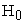and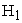were used here too.

Data were then simulated underthree different sample sizes, 10, 15 and 20 respectively. In SAS, PROC GENMOD facilitates the GEE method and it is available under SAS version 9.0 while GLMMs methods which is facilitated by PROC GLIMMIX is available only in the versions of SAS 9.2 or later. For each combination of the respective method and available sandwich variance modifications simulations were carried out 1000 times.

From the results obtained, averaged estimated probabilities under each period, the proportion of rejections ofwhenis true (Type 1 Error) and whenis true (power), number of non-convergent results and average variance of the period estimates were obtained. The change in percentage of estimated variance over true variance was calculated from the output. The significance level used in the study is 5%. The three methods were compared with respect to the above mentioned results obtained from the simulation study.

### 3. Methodology

3.1. Generalized Estimating Equations Method

This approach, which is an extension of Generalized Linear Models (GLM) was introduced by 5; for longitudinal data to estimate the population averaged estimates by considering correlation between repeated measurements. Here, the mean response and within the subject association is modelled separately and the parameters in this model explain the variation of mean response over time 6.

Suppose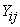(where j=1,…,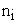and i=1,…,m) represents the jth measurement on the ith subject and there are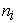measurements on subject i. Hence the total number of measurements is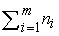.

Hence the marginal regression model is given in equation (1).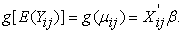(1)

This is similar to the systematic Component where,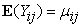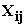– p x 1 vector of study variables (covariates) for the ith subject

g (.) – Link function that relates the means of the responses to the linear predictor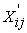β

β – Vector of p regression parameters to be estimated.

Reference 3 has given a detailed account of this method for the general case of sample size. Here for the special case of small sample size, 3 showed through simulation that there was bias in the estimation. Therefore, here we propose to use the Sandwich Variance Estimator of the parameter estimates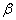as a correction for this bias.This is explained in section 3.1.1.

3.1.1. Estimating the Covariance of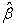1. Model Based (“Naive”) Covariance Estimator is given by Eq. (2),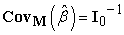(2)

Where,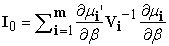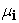– Vector of mean of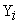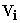– Modeled covariance matrix of.

This is a consistent estimator for the covariance matrix ofas long as both mean model and the working correlation matrix are specified correctly.

2. Empirical (“Robust”) Covariance Estimator is given by Eq. (3),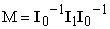(3)

Where,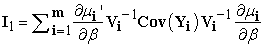Cov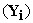– True covariance matrix ofThis estimator was proposed by 5 and it is consistent for the Cov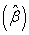as the number of clusters become large and valid even if the working correlation matrix is not specified correctly 12.

3.2. Generalized Linear Mixed Models (GLMMs)

Generalized Linear Mixed Models (GLMMS) is an extension of the Generalized Linear Model (GLM) as the linear predictor incorporates random effects in addition to the fixed effects 2. Hence the model is as in Eq. (4),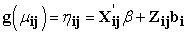(4)

Where,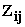– 1 x q vector of study variables (covariates) for the ithsubject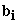– q x 1 vector of random effects.

Reference 3 has explained this model in detail. Thus, as in section 3.1 an explanation is only given of the Sandwich Variance Estimation methods which are used to control small sample bias.

3.2.1. Sandwich or Empirical Covariance Estimators

Residual-Based Estimators

The general form of the empirical covariance estimator with the small sample bias corrections (multiplicative and additive) can be written as equation (5),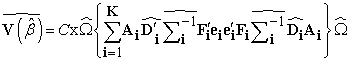(5)

Where, E(Y) =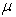,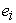=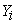-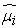, Var (Y) =∑ , D =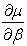,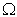=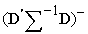.

Here m is the number of independent sampling units and this estimator is biased if m is small. All the bias correction methods vary with different combinations of values applied for the constant C and the matrices of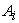and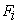. Table 1 displays the form of multiplicative small sample bias corrections applied to the sandwich estimator available with PROC GLIMMIX in SAS 9.

Here,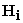=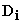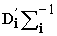, Q =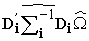, k-The rank of X matrix and r is an optional number 0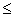r <1 is a constant chosen to provide an upper bound on the correction factor (Default r = 0.75).

Likelihood Based Estimators

1. Classical Sandwich Estimator is given by Eq. (6),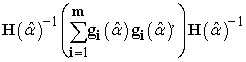(6)

Where,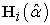is the Second derivative of the log likelihood for the ith subject derived for some parameter vector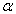,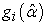is the First derivative of the log likelihood for the ith subject and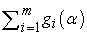is the Gradient for the entire data 9.

2. MBN Adjustment is given by Eq. (7),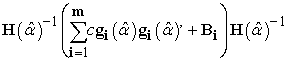(7)

Where,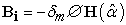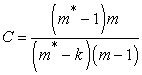where m* number of observations and m number of subjects.

Also,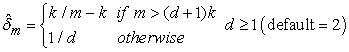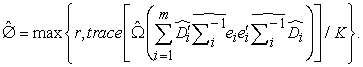Note: Reference 3 explains the desirable properties of estimators in detail.

### 4. Results from the Simulation Study

The results obtained from the simulation study are presented in this section and a broad explanation is made on these. The following data is comprised of important results obtained under simulation studies conducted using varying options of the SVE techniques available in GENMOD and in GLMM in SAS. In the presentation using tables only the results from SAS procedures and SVE methods, giving satisfactory modes of analyzing small sample BRM is presented due to space limitations. However, all results (even those not presented in tables) are explained using text and tables of all results are presented in the supplementary material.

Parameters were estimated using the GEE method and GLMMs which were facilitated by PROC GENMOD and PROC GLIMMIX procedures in SAS. Each combination of method and respective SVE technique were simulated 1000 times under null and alternative hypothesis using three different sample sizes of 10, 15 and 20. Data were simulated in a way that the data were correlated to apply the repeated binary structure in the data 3. Since three periods were considered three repeated binary observations were simulated for each observation. The properties of the parameter estimates generated under each option was used to satisfy the objectives.

4.1. Initiation
4.1.1. Hypothesis of Interest: All period effects are equal: At least one effect is significantly different from others

4.1.2. Initial Probabilities

Initial probabilities assigned for three different scenarios are as given in 3. This is given in Table 2.

4.2. Results from the PROC GENMOD Procedure for GEE Method in SAS
4.2.1. Tables of Results

This section interprets the results of two approaches of estimation of parameters obtained from PROC GENMOD procedure in SAS for binary RMD with small sample sizes. The results are measured using model based variance covariance matrix while under the other approach the results are generated by using Sandwich Variance covariance matrix.

Under each option, the number of non-convergent results, the proportion of rejections of, averaged estimated probability and estimated variance for each period averaged over 1000 simulations, bias and change in percentage variances were computed. Table 3 gives Results from the GEE method of PROC GENMOD with no adjustments (Naive Method). The results from the GEE method of PROC GENMOD with the SVE adjustment were obtained but not presented here. Table 4 gives a comparison of these two methods. Table 3 represents the results obtained from the GEE method under PROC GENMOD procedure in SAS relying on the model based standard errors (naive method) with exchangeable correlation structure. According to Table 3 the Type I error only for sample size 10 lies within the stipulated 95% probability interval for error rate of 5% with 1000 simulations [0.036, 0.064] while the Type I error of other two samples are conservative. It is also noticeable that the Type I error decreases with the increment of sample size. With respect to, the power increases with the sample size. The results also depict that no non-convergence issues occur in the GEE method of PROC GENMOD procedure with naive method under both null and alternative hypotheses. In this method the average predicted probability values are closer to the true values under both hypotheses for all sample sizes, indicating that the unbiasedness of estimates are achieved for every sample size under both hypotheses. It is worth noting that the parameter estimates are more unbiased under this method compared to GLMM method with any of the SVE techniques. The average variance under both hypotheses strictly decrease when the sample size increases. Due to the consideration of the average variance of the estimated values as a measure of variability, decrement in this value with sample size can be used to validate the property of consistency of parameter estimates derived by the GEE method of PROC GENMOD with no adjustments. When considering the property of sufficiency, the percentage difference between estimated and true variance of period effect are very small under the null and alternative hypotheses. These values are smaller than those given by GLMM with a variety of SVE techniques. Hence this method produces the most sufficient estimated period effects compared to GLMM method 3. As expected the time to compute the results increases with sample size under both hypotheses.

The performance of the test and the properties of the parameter estimates corresponding to the GEE method facilitated by PROC GENMOD procedure with the robust variance estimation method was examined but not presented. The GEE adjustment has influenced the results of the ordinary GEE method by inflating Type I error of all sample sizes. Though the power of the study has a significant improvement, since the most important property considered, Type I error is violated, this adjustment is not suitable. Hence the performance of other properties has not been discussed in detail.

4.2.2. Comparison of varying options of GEE method in SAS

Table 4 gives a comparison of the methods examined under GEE.

Table 4 makes an overall comparison among the varying options of Generalized Estimating Equation methods available under PROC GENMOD in SAS. Both methods perform well with respect to the properties of unbiasedness, sufficiency and consistency of the parameter estimates.

With respect to other properties, except for Type I error, GEE method without the robust variance estimation (naive) method performs well as the estimates do not encounter with non-convergence issues and the speed of computation is highest. On the other hand the GEE method with robust variance estimation method performs well with respect to power and generates more efficient estimates but has poor performance towards other properties. Since this most important property considered in the selection of a suitable method is violated, this adjustment is not suitable. The power of the study has a significant improvement.The GEE modification has brought up the issue of non-convergence. The property of unbiasedness is achieved in magnitude, under both hypotheses for all sample sizes. It is also easily identified that with respect to the property unbiasedness, the best estimates are given by GEE method of PROC GENMOD. Consistent parameter estimates are given.

Overall, it can be concluded that the estimated period effects are sufficient. It is also confirmed that with respect to the property sufficiency, best parameter estimates are generated by the two options available in GEE method compared to GLMM method of PROC GLIMMIX with all possible SVE modifications. The time taken to generate the results increases as the sample size increases under both hypotheses. When comparing the overall average variance of the estimated probabilities, both methods provide values which are almost equal to each other. But a pairwise comparison, depicts that the GEE method with robust variance estimation method provides the more efficient parameter estimates.

4.3. Results from the PROC GLIMMIX Procedure for GLMMs Method in SAS
4.3.1. Tables of Results

This subsection discusses the results obtained from the PROC GLIMMIX procedure in SAS which estimates the parameters of the GLMMs method (naive) and gives the results obtained by improving the properties of the type III F tests using the Sandwich Variance Modifications available in SAS such as, Classical, DF, ROOT, FIRORES, FIROEEQ and MBN. Under each option important properties of parameter estimates were computed and estimated variance was computed for each period averaged over 1000 simulations. Only the results for the naive method and SVE methods, ROOT and FIROEEQ are presented here in Table 5, Table 6 and Table 7. As there are many methods the results are not given under each table, but summarized at the end of all the GLMM methods. Table 5 consists of results obtained from the GLMMs simulated using Quadrature method, without making any adjustments to the covariance matrix of the parameter estimates (Naive-method). Table 6Table 7 give the results for the SVE techniques ROOT and FIROEEQ respectively.

4.3.2. Comparison of Varying Options of GLMM Method in SAS

Table 8 gives Comparison of varying options of GLMM method in SAS. Table 8 makes a straightforward comparison between available options of GLMM methods in PROC GLIMMIX with respect to the properties of the parameter estimates. It’s clear that none of the methods are suitable with respect to the properties convergence and sufficiency. Also the parameter estimates are consistent and unbiased irrespective of the method used. Hence, these three properties do not assist the selection of the most suitable method. When comparing the methods with the rest of the properties it can be identified that both FIROEEQ and ROOT options perform well. The reasons leading to this conclusion are that Type I error holds for both methods under all sample sizes and the magnitude of the power is considerably high for both methods. The most efficient estimates are given by the FIROEEQ option followed by ROOT. Even in speed of computation methods FIROEEQ perform well. Due to the positive response towards the majority of the parameter estimates, this leads to conclude that with respect to the GLMM procedure, PROC GLIMMIX with FIROEEQ option performs best, followed by the ROOT option and these are suitable to analyze BRM data with small sample sizes.

### 5. Discussion

5.1. Introduction

The majority of the studies conducted to analyze BRM data was based on large sample sizes. Hence the prime objective of this study was to recommend a method to analyze binary RMD with small sample size. Since the variance of the parameter estimates tends to be biased as the sample size reduces, SVE techniques available under each method were taken into account as a remedial measure. Hence two methods available with SVE techniques, namely, Generalized Estimating Equations (GEE) method and Generalized Linear Mixed Models (GLMMs) were used in this study. The GEE method represents marginal or population averaged models and GLMM method use random-effect or subject-specific models. In SAS software PROC GENMOD facilitates GEE method from version 9.0 onwards and PROC GLIMMIX facilitates GLMM method which is available only in SAS versions from 9.2. In order to achieve the ultimate goal, a large scale simulation study was carried out in SAS. Correlated binary observations were generated under three different small sample sizes (10, 15 and 20) with three time points while thousand simulations were carried out underandfor each method along with available SVE techniques in SAS. The properties convergence, Type I Error, power, unbiasedness, consistency, sufficiency, and efficiency were then computed along with the speed of computation under each procedure and proceeded with comparison of each method.

5.2. Important Findings from the Simulation Study

Under each combination of binary RMD analysis method and available SVE techniques variety of properties of parameter estimates were considered. Among these properties Type I error and power were of major interest and the results were drawn mainly on the performance made on these two properties.

5.2.1. Findings from the PROC GENMOD Procedure for GEE Method

The two varying options considered under this method were the naive approach and the Robust Variance Estimation method. When considering the results generated under PROC GENMOD procedure with Robust Variance Estimation method, though some properties of parameter estimates hold, it was unable to generate Type I error for any of the sample sizes being tested and the estimated type I error deviated from lying within the stipulated 95% probability interval [0.036, 0.064]. Hence this option cannot be recommended to analyze binary RMD with small sample sizes as the property Type I error, which is one of the most important factors considered in this study was not satisfactory.

However the property Type I error is satisfied by the PROC GENMOD procedure without imposing a variance adjustment (naive method) for very small sample sizes. Hence it is worthwhile to look into other significant properties satisfied by this method.

Properties of PROC GENMOD procedure without imposing a variance adjustment (naive method)

Type I error barely hold for very small sample sizes (lie on the lower limit of the stipulated confidence interval for Type I error). The power of the test; the proportion of rejections ofwhenis true, increases with sample size. There are no non-convergence issues for any sample size. Since estimated probability values both underandare closer to true probability values for three samples, it conveys the property of unbiasedness. Average variance decreases as sample size increases under both hypotheses implies consistency of the estimates. The percentage difference between the estimated and true variance of period effects being significantly low suggests sufficiency of the estimates. Time to compute the results increases with sample size under both hypotheses.

5.2.2. Findings from the PROC GLIMMIX Procedure for GLMM Method

Seven varying options were considered under the PROC GLIMMIX procedure for GLMM method, namely, naive approach, Classical Sandwich, DF, ROOT, FIRORES, FIROEEQ and MBN.

Among these options only the PROC GLIMMIX procedure with ROOT and FIROEEQ were capable of generating results such that Type I error lie within the 95% probability interval for an error rate of 5%=0.05 for 1000 samples that is [0.036, 0.064]. Hence none of the other options of PROC GLIMMIX procedure apart from these two adjustments can be recommended to analyze binary RMD with small sample size. It is important to note that these two options satisfy the property of Type I error for all three sample sizes. Hence this highly influences the selection criteria. It is of major interest to look towards the other properties being satisfied.

Properties of PROC GLIMMIX procedure with the SVE method- ROOT

Type I error values all lie within the 95% stipulated limits [0.036, 0.064]. Power increases with the sample size. Since estimated probability values both underandare closer to true probability values for three samples, the property of unbiasedness holds. Average variance decreases as sample size increases under both hypotheses suggests consistency of the estimates. Time to compute the results increases with sample size under alternative hypothesis and time taken for computation is considerably small.

Properties of PROC GLIMMIX procedure with the SVE method- FIROEEQ

Type I error under all 3 sample sizes lie within the 95% stipulated limits [0.036, 0.064]. Power increases as the sample size increases. Since estimated probability values both underandare closer to true probability values for three samples, it conveys the property of unbiasedness. The average variance decreases as sample size increases under both hypotheses suggest consistency of the estimates. Time to compute the results increases with sample size under alternative hypothesis and time taken for computation is as small as in the ROOT option.

5.3. Conclusions

Under both methods irrespective of the SVE technique used, all estimates satisfy unbiasedness and consistency. GLMM with both SVE techniques FIROEEQ and ROOT showcase outstanding performances in analyzing small sample binary RMD with respect to all the properties of the parameter estimates except for the property of sufficiency. However, if one is more concerned about the property sufficiency, GEE method with no variance adjustment (naive method) is recommended for very small sample sizes. All these results are applicable to BRM data with small sample sizes which adhere to AR (1) process.

This study was conducted for BRM data with no missing values (balanced data), with no covariates and with a limited number of time points. When considering varying options available in GEE method, it is evident that GEE method with no variance adjustments imposed (naive method) has outstanding performances when the sample size is very small with no missing values and no covariates. Under GLMM method the performance is significantly improved once the small sample multiplicative adjustments, FIROEEQ and ROOT are introduced to the model. Except for sufficiency, these two methods can be recommended for a scenario of small sample size binary repeated measurement case.

However, due to the time constraint the default options of each SVE technique were considered and asymptotic chi-square distribution (default in SAS) was used to compute the critical values. Also the study was restricted to binary RMD and couldn’t extend to categorical RMD.

Since, this study only focused on binary RMD and the scope of the study can be extended into following aspects. Analyze RMD with categorical (nominal and ordinal) response variables. Extend this study to include missing values and their imputations. Analyze RMD with the presence of time variant and time in-variant covariates. Since this study considered only period effects, this study can be extended by taking different covariates such as categorical, continuous into account.

### Acknowledgements

I would like to extend my sincere thanks to all the lecturers of the Department of Statistics, University of Colombofor supporting in numerous ways.

### References

  Fitzmaurice, G., Davidian, M., Verbeke, G. and Molenberghs, G, Longitudinal Data Analysis, CRC Press, 2008, 3-27. In article View Article  Fong, Y., Rue, H. and Wakefield, J, “Bayesian inference for generalized linear mixed models,” Biostatistics, 11(3). 397-412. Dec.2009. In article View Article  PubMed  Gawarammana, M. B. and Sooriyarachchi, M. R, “Comparison of methods for analyzing binary repeated measures data: A Simulation Based Study (comparison of methods for binary repeated measures),”Communications in Statistics - Simulation and Computation, 46(3). 2103-2120. May.2015. In article View Article  Lalonde, T. L., Nguyen, A. Q., Yin, J., Irimata, K. and Wilson, J. R, “Modeling Correlated Binary Outcomes with Time-Dependent Covariates,” Journal of Data Science, 11(4). 715-738. Oct .2013. In article View Article  Liang, K.Y. and Zeger, S. L, “Longitudinal data analysis using generalized linear models,” Biometrika, 73(1). 13-22. Apr.1986. In article View Article  Locascio, J. J. and Atri, A, “An Overview of Longitudinal Data Analysis Methods for Neurological Research,” Dementia and geriatric cognitive disorders extra, 1(1). 330-357. Oct.2011. In article View Article  PubMed  Mahan, V. L, “Clinical Trial Phases,” International Journal of Clinical Medicine, 5(21). 1374-1383. Dec.2014. In article View Article  Ramezani, N, “Analyzing Correlated Data in SAS®,” in SAS® GLOBAL FORUM 2017, 1251. In article  SAS Institute Inc, SAS/STAT® 9.2 User's Guide, 2008. [E-book] Available: open e-book. In article  Sunethra, A. A. and Sooriyarachchi, M. R, “Sandwich Variance Estimation for random effect misspecification in Generalized Linear Mixed Models,” GSTF Journal of Mathematics, Statistics and Operations Research (JMSOR), 3(2). 8-11. Jul.2016. In article View Article  Szmaragd, C., Clarke, P. and Steele, F, “Subject specific and population average models for binary longitudinal data: a tutorial,” Longitudinal and Life Course Studies, 4(2). 147-165. May 2013. In article View Article  Wang, M, “Generalized Estimating Equations in Longitudinal Data Analysis: A Review and Recent Developments,” Advances in Statistics, 2014. Dec.2014. In article View ArticleThis work is licensed under a Creative Commons Attribution 4.0 International License. To view a copy of this license, visit http://creativecommons.org/licenses/by/4.0/

### Cite this article:

##### Normal Style
Dharmaratne ADVTT, Sooriyarachchi MR. Methods for Analyzing Binary Repeated Measures: The Small Sample Case. American Journal of Applied Mathematics and Statistics. Vol. 5, No. 2, 2017, pp 80-89. http://pubs.sciepub.com/ajams/5/2/6
##### MLA Style
ADVTT, Dharmaratne, and Sooriyarachchi MR. "Methods for Analyzing Binary Repeated Measures: The Small Sample Case." American Journal of Applied Mathematics and Statistics 5.2 (2017): 80-89.
##### APA Style
ADVTT, D. , & MR, S. (2017). Methods for Analyzing Binary Repeated Measures: The Small Sample Case. American Journal of Applied Mathematics and Statistics, 5(2), 80-89.
##### Chicago Style
ADVTT, Dharmaratne, and Sooriyarachchi MR. "Methods for Analyzing Binary Repeated Measures: The Small Sample Case." American Journal of Applied Mathematics and Statistics 5, no. 2 (2017): 80-89.
Share